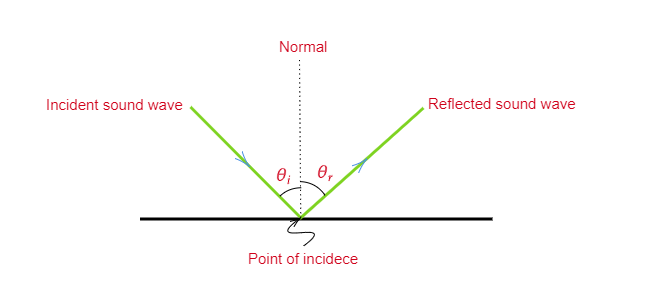# Does sound follow the same laws of reflection as light does? Explain.

Yes, sound follows the same laws of reflection as light. let us discuss what is the reflection of sound and what laws does the reflection of a sound wave are followed:

Reflection of sound:
When a sound bounces back from any surface, it is known as the reflection of the sound. And hard surfaces are best for reflecting the sound waves.

Examples of the reflection of the sound:
$(i)$. Working process of SONAR- The speed and distance of underwater objects is measured by using the reflection of sound. This method is called SONAR.

$(ii)$. Working on a stethoscope-the sound of a patient’s heartbeat reaches the doctor’s ear through multiple reflections of sound.

Laws of reflection of sound:
The following laws the reflection of a sound wave follows:

1. The reflected sound wave and the incident sound wave make an equal angle with the normal to the surface at the point of incidence.

2. The reflected sound wave, the normal to the point of incidence, and the incident sound wave all lie in the same plane.

The diagram given below shows the reflection of sound.